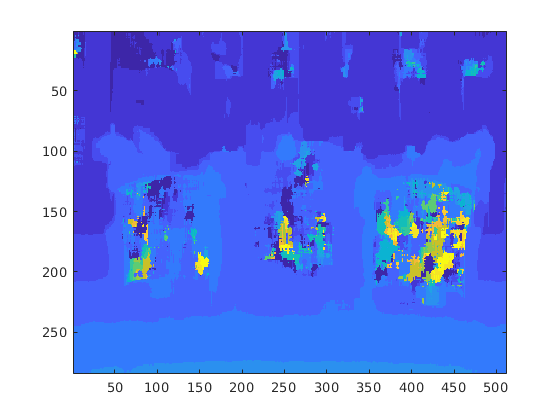# Stereo Disparity

This example shows how to generate a CUDA® MEX function from a MATLAB® function that computes the stereo disparity of two images.

### Third-Party Prerequisites

Required

This example generates CUDA MEX and has the following third-party requirements.

• CUDA enabled NVIDIA® GPU and compatible driver. For half-precision code generation, the GPU device must have a minimum compute capability of 6.0.

Optional

For non-MEX builds such as static, dynamic libraries or executables, this example has the following additional requirements.

### Verify GPU Environment

To verify that the compilers and libraries necessary for running this example are set up correctly, use the `coder.checkGpuInstall` function.

```envCfg = coder.gpuEnvConfig('host'); envCfg.BasicCodegen = 1; envCfg.Quiet = 1; coder.checkGpuInstall(envCfg);```

### Stereo Disparity Calculation

The `stereoDisparity.m` entry-point function takes two images and returns a stereo disparity map computed from the two images.

`type stereoDisparity`
```%% Modified Algorithm for Stereo Disparity Block Matching % In this implementation, instead of finding shifted image, indices are % mapped accordingly to save memory and some processing. RGBA column major % packed data is used as input for compatibility with CUDA intrinsics. % Convolution is performed using separable filters (horizontal and then % vertical). % Copyright 2017-2021 The MathWorks, Inc. function [out_disp] = stereoDisparity(img0,img1) %#codegen % Copyright 2017-2019 The MathWorks, Inc. % GPU code generation pragma coder.gpu.kernelfun; %% Stereo Disparity Parameters % |WIN_RAD| is the radius of the window to be operated. |min_disparity| is % the minimum disparity level the search continues for. |max_disparity| is % the maximum disparity level the search continues for. WIN_RAD = 8; min_disparity = -16; max_disparity = 0; %% Image Dimensions for Loop Control % The number of channels packed are 4 (RGBA) so as nChannels are 4. [imgHeight,imgWidth]=size(img0); nChannels = 4; imgHeight = imgHeight/nChannels; %% Store the Raw Differences diff_img = zeros([imgHeight+2*WIN_RAD,imgWidth+2*WIN_RAD],'int32'); % Store the minimum cost min_cost = zeros([imgHeight,imgWidth],'int32'); min_cost(:,:) = 99999999; % Store the final disparity out_disp = zeros([imgHeight,imgWidth],'int16'); %% Filters for Aggregating the Differences % |filter_h| is the horizontal filter used in separable convolution. % |filter_v| is the vertical filter used in separable convolution which % operates on the output of the row convolution. filt_h = ones([1 17],'int32'); filt_v = ones([17 1],'int32'); % Main Loop that runs for all the disparity levels. This loop is % expected to run on CPU. for d=min_disparity:max_disparity % Find the difference matrix for the current disparity level. Expect % this to generate a Kernel function. coder.gpu.kernel; for colIdx=1:imgWidth+2*WIN_RAD coder.gpu.kernel; for rowIdx=1:imgHeight+2*WIN_RAD % Row index calculation. ind_h = rowIdx - WIN_RAD; % Column indices calculation for left image. ind_w1 = colIdx - WIN_RAD; % Row indices calculation for right image. ind_w2 = colIdx + d - WIN_RAD; % Border clamping for row Indices. if ind_h <= 0 ind_h = 1; end if ind_h > imgHeight ind_h = imgHeight; end % Border clamping for column indices for left image. if ind_w1 <= 0 ind_w1 = 1; end if ind_w1 > imgWidth ind_w1 = imgWidth; end % Border clamping for column indices for right image. if ind_w2 <= 0 ind_w2 = 1; end if ind_w2 > imgWidth ind_w2 = imgWidth; end % In this step, Sum of absolute Differences is performed % across tour channels. tDiff = int32(0); for chIdx = 1:nChannels tDiff = tDiff + abs(int32(img0((ind_h-1)*(nChannels)+... chIdx,ind_w1))-int32(img1((ind_h-1)*(nChannels)+... chIdx,ind_w2))); end % Store the SAD cost into a matrix. diff_img(rowIdx,colIdx) = tDiff; end end % Aggregating the differences using separable convolution. Expect this % to generate two kernels using shared memory.The first kernel is the % convolution with the horizontal kernel and second kernel operates on % its output the column wise convolution. cost_v = conv2(diff_img,filt_h,'valid'); cost = conv2(cost_v,filt_v,'valid'); % This part updates the min_cost matrix with by comparing the values % with current disparity level. for ll=1:imgWidth for kk=1:imgHeight % load the cost temp_cost = int32(cost(kk,ll)); % Compare against the minimum cost available and store the % disparity value. if min_cost(kk,ll) > temp_cost min_cost(kk,ll) = temp_cost; out_disp(kk,ll) = abs(d) + 8; end end end end end ```

### Read Images and Pack Data Into RGBA Packed Column-Major Order

```img0 = imread('scene_left.png'); img1 = imread('scene_right.png'); [imgRGB0] = pack_rgbData(img0); [imgRGB1] = pack_rgbData(img1);```

### Left Image### Right Image### Generate GPU Code

```cfg = coder.gpuConfig('mex'); codegen -config cfg -args {imgRGB0, imgRGB1} stereoDisparity;```
```Code generation successful: View report ```

### Run Generated MEX and Show the Output Disparity

```out_disp = stereoDisparity_mex(imgRGB0,imgRGB1); imagesc(out_disp);```### Half Precision

Computations in this example can also be done in half-precision floating point numbers, using the stereoDisparityHalfPrecision.m entry-point function. To generate and execute code with half-precision data types, CUDA compute capability of 6.0 or higher is required. Set the `ComputeCapability` property of the code configuration object to `'6.0'`. For half-precision, the memory allocation (malloc) mode for generating CUDA code must be set to 'Discrete'.

```cfg.GpuConfig.ComputeCapability = '6.0'; cfg.GpuConfig.MallocMode = 'Discrete';```

The standard `imread` command represents the RGB channels of an images with integers, one for each pixel. The integers range from 0 to 255. Simply casting inputs to half type might result in overflow during convolutions. In this case, we can scale the images to values between 0 and 1. "imread" represents the RGB channels of an images with integers, one for each pixel. The integers range from 0 to 255. Simply casting inputs to half type might result in overflow during convolutions. In this case, we can scale the images to values between 0 and 1.

```img0 = imread('scene_left.png'); img1 = imread('scene_right.png'); [imgRGB0] = half(pack_rgbData(img0))/255; [imgRGB1] = half(pack_rgbData(img1))/255;```

### Generate CUDA MEX for the Function

Code generation on the `stereo_disparity_half_precision.m` function.

`codegen -config cfg -args {imgRGB0, imgRGB1} stereoDisparityHalfPrecision;`
```Code generation successful: View report ```